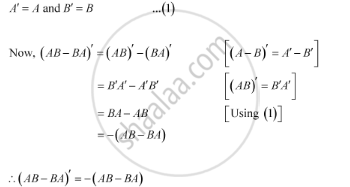Share

# If a and B Are Symmetric Matrices, Prove that Ab − Ba is a Skew Symmetric Matrix - CBSE (Commerce) Class 12 - Mathematics

ConceptSymmetric and Skew Symmetric Matrices

#### Question

If A and B are symmetric matrices, prove that AB − BA is a skew symmetric matrix

#### Solution

It is given that A and B are symmetric matrices. Therefore, we have:Thus, (AB − BA) is a skew-symmetric matrix.

Is there an error in this question or solution?

#### Video TutorialsVIEW ALL 

Solution If a and B Are Symmetric Matrices, Prove that Ab − Ba is a Skew Symmetric Matrix Concept: Symmetric and Skew Symmetric Matrices.
S